Next: Inversive Congruential Generators Up: Random Number Generators Previous: Random Number Generators

## Linear Congruential Generators

Linear Congruential Generators (LCG) are one of the oldest and most studied RNGs . A LCG is parameterized by three integers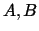, and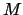. Its basic form is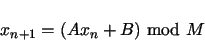There are two characteristics of LCGs:

• Periodicity. Given an initial seed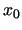, there is some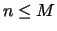such that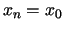. We say the periodicity of this LCG is the least such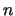. The existence of period can be proved by a simple application of the pigeonhole principle.

• Parallel Hyperplanes (Lattices). When we plot the set of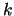-dimensional points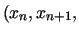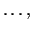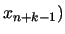(for all) in-dimensional space, we will observe that all points fall mainly in the hyperplanes. There are actually more than one set of parallel hyperplanes if seeing the-dimensional space from a different orientation. Figures 1 and 2 illustrate this phenomenon.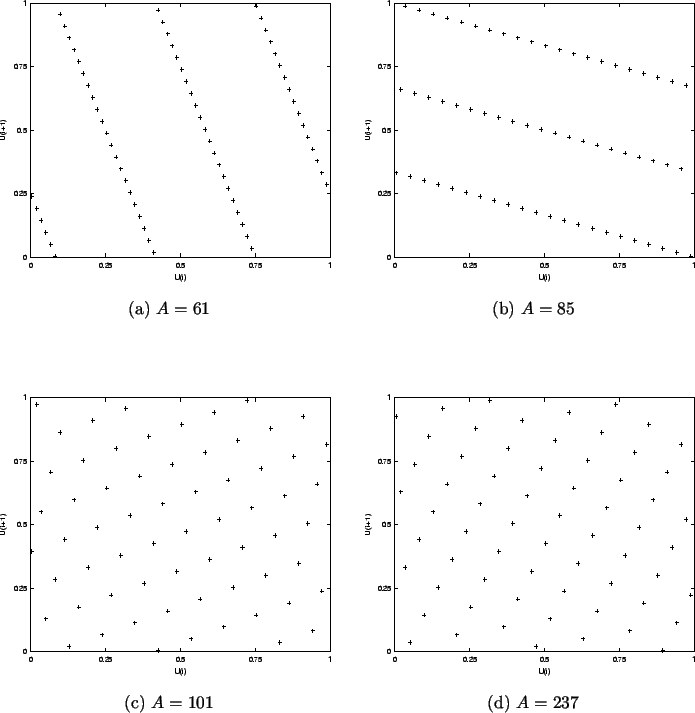[scale=0.7]figures/randu.eps

A LCG has full period if its period length is. There are several conditions for an LCG to reach full period. For example,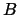andmust be relatively prime; any prime dividesmust also divide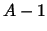. Long period is easy to achieve but is not everything, because LCGs with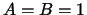is perfectly 1-distributed, but they have terrible statistical properties like extremely high autocorrelation.

Another requirement for a good LCG is to minimize the parallel hyperplanes'' phenomenon . Let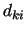be the distance between two parallel hyperplanes seen from orientation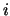. Then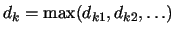is the-dimensional maximum distance between two parallel hyperplanes from all orientations. The search for optimal parameters that minimizes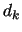thus becomes the most important issue for LCGs. Fortunately, one test called Spectral test is designed to quickly calculategiven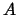,, and.

The generalized congruential generators have the following form (see ):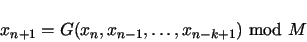For example, a quadratic congruential generator has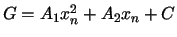. If linearity must be maintained, then we can take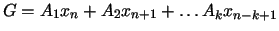. This kind of generator is called multiplicative recursive generator (MRG). A restricted form of MRG called Fibonacci generator has only two of the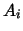coefficients being nonzero. These variants may have longer periods and good statistical properties, but it is more complicated to assess their periodicities and randomness based on their parameters.

In this project we have implemented a special kind of LCG called Prime Modulus Multiplicative Linear Congruential Generator (PMMLCG.) Its parameters are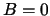andbeing a prime. The advantage of PMMLCG is that it eliminates an addition, has an almost full period (of length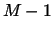), and can be subjected to the Spectral test.Next: Inversive Congruential Generators Up: Random Number Generators Previous: Random Number Generators
2001-05-30